# Electronics and Communication Engineering - Exam Questions Papers

6.

The impulse response of an initially relaxed linear system is e-2tu(t) . To produce a response te-2tU(t), the input must be equal to __________ .

 A. e-2t U(t) B. e-lt U(t) C. e-1u(t) D.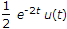Explanation:

Given, h(t) = e-2tu(t) H(s) =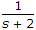y(t) = te-2t, u(t) y(s) =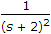Using Laplace Transform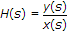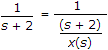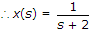x(t) = e-2tu(t)

Input must be equal to e-2tu(t).

7.

The continuous time system with impulse response h(t)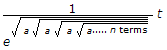u(t) is stable, if __________ (n is even).

 A. a = 0 B. a > 0 C. a < 0 D. none of these

Explanation:

h(t) =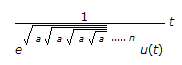consider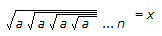ax = x

ax = x2

a = x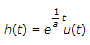For h(t) to be bounded, exponential term should have a negative power.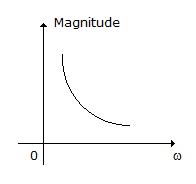i.e. e-t will have response as shown.

Hence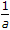should be < 0

a < 0.

8.

Consider the following diagrams showing vector fields :
The correct statements are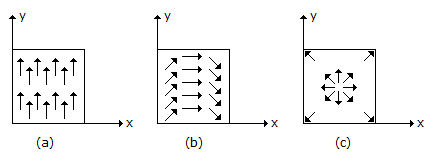A. For figure (a), ∇ .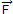= 0, ∇ x= 0 B. For figure (a), ∇ .= 0, ∇ x≠ 0 C. For figure (b), ∇ .= 0, ∇ x≠ 0 D. For figure (c), ∇ .= 0, ∇ x≠ 0

Explanation:

In figure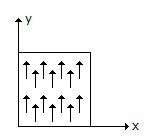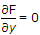Fx = 0 hence div F = ∇ .= 0, but curl F ≠ 0.

In figure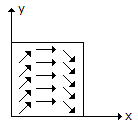magnitude of F remains constant and hence ∇ .= 0 and also it is possible that ∇ .= 0, as there can be an electrostatic field in which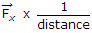and circulation around any closed path in it may be equal to zero.

In Figure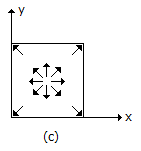has a central field (radial).

∇ x= 0, ∇≠ 0.

9.

In the signal flow graph of figure, y/x equals.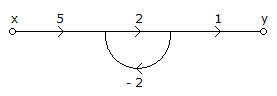A. 3 B. 5/2 C. 2 D. None of the above

Explanation:

P1 = 5 x 2 x 1 = 10

L1 = - 4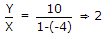.

10.

Find resistance RB to bring transistor to threshold of saturation (VCB = 0), VBE = 0.7 V, a = 0.96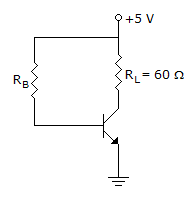A. 1.5 KΩ B. 15 KΩ C. 10 KΩ D. 1 KΩ

Explanation: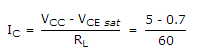= 0.07 A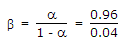= 24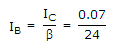= 3 mA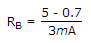= 1433.33 Ω ≅ 1.5 KΩ.

#### Current Affairs 2022

Interview Questions and Answers